Zen Pharaohs of Ultimate Love mathematics, music, science, wood....what else could you ask?Next: Recursive Implementation of General Up: Recursive Windowing of Time Previous: Recursive Windowing of Time

# Recursive Windowing of Time Series

Windowing a time series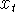means computing another time series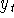by the formula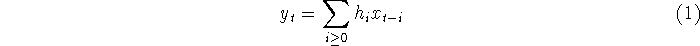where the numbers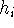define the window. In the case where the input seriesis a unit impulse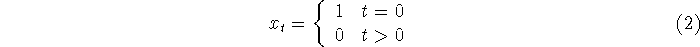the output time seriesis called the impulse response of the windowing filter. Alternatively, we can obtain the seriesby passingthrough any filter with the same impulse response. Different filters with the same impulse response are called different realizations of each other. Although all realizations of the a filter have the same signal characteristics, not all realizations of the same filter have the same properties when approximated by finite precision (fixed or floating point) arithmetic, and not all realizations require the same amount of computational cycles. This note explains how to obtain particularly advantageous realizations.

One practical difficulty in using the representation (1) is that it requires storage of all the values of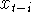for which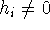, and when used sequentially, in storage of all the values of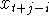for which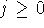and. In particular, it is not practical to realize an IIR (infinite impulse response) filter in this way.

The most common IIR impulse response used for windowing is the case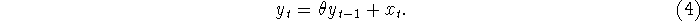It is frequently useful to refer to the DC gain of the filter, which is the sum of the impulse response. In this case, the DC gain is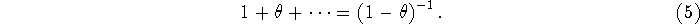Another filter which is used for windowing is the `modified Barnwell' window suggested by Strobach. This second order filter generalizes (4) is given by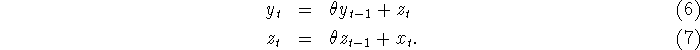The impulse response of this window is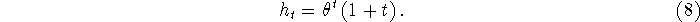It is a little easier to see this if we recast the filter in the state space form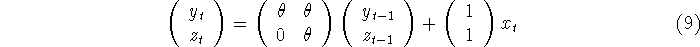so that the impulse response is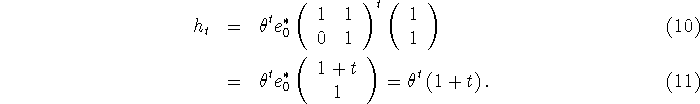Note that we are numbering the indices of the vector starting at 0, hence the first standard basis vector is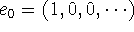, etc.

The DC gain is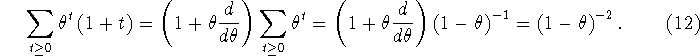Next: Recursive Implementation of General Up: Recursive Windowing of Time Previous: Recursive Windowing of Time

Fri Jun 27 03:10:38 EDT 1997